# The rod

The rod is painted in four colors. 55% of the bar is painted in blue, green 0.2 of the rod, 1/8 is brown, and the remaining 45 cm of white. How long is the rod?

x =  360 cm

### Step-by-step explanation:

x-55/100x - 0.2 x - 1/8x = 45

x-55/100•x - 0.2•x - 1/8•x = 45

25x = 9000

x = 360

Our simple equation calculator calculates it.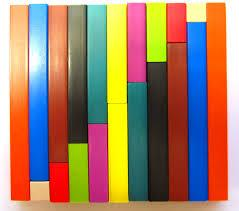Did you find an error or inaccuracy? Feel free to write us. Thank you!Tips to related online calculators
Need help to calculate sum, simplify or multiply fractions? Try our fraction calculator.
Do you have a linear equation or system of equations and looking for its solution? Or do you have a quadratic equation?

## Related math problems and questions:

• Bar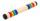Half of the bar is green, the third is yellow and the remaining 40cm is red. How long is a bar?
• The thirdThe one-third rod is blue, one-half of the rod is red, the rest of the rod is white and measures 8 cm. How long is the whole rod?
• PC disksPeter has 45 disks in three colors. One-fifth of the disks are blue, red are twice more than the white. How much is blue, red and white disks?
• Alcohol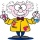6l 48% alcohol, how much 52% alcohol must be added to give 50% alcohol?
• Above 100%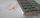Find 340 percent of the numbers 45 and 55.
• The ratio 4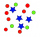The ratio of two number is 5:4 if 40% of the first number is 12, what will be 50% of the second number?
• Three glasses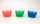Three glasses of different colors have different volumes. Red 1.5 liter is filled from 2/5, blue 3/4 liter is filled from 1/3, and the third green 1.2 liter is empty. Pour green glass 1/4 of the contents from the red glass and 2/5 of the content from the
• Four numbersThe first number is 50% second, the second number is 40% third, the third number is 20% of the fourth. The sum is 396. What are the numbers?
• The rodThe rod has a length of 90cm. Half is painted blue, the third is red and the rest is yellow. How long is the yellow part of the rod?
• Here are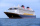Here are white, blue, and red boats in a marina. Two-thirds of the boats in the marina are white, 4/7 of the remaining boats are blue, and the rest are red. If there are 15 red boats, how many boats are in the marina? Which tape diagram represents how man
• Percent calculationCalculate 8% if 44% is 32.
• Vinegar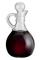We must dilute 16 liters of 8.8% aqueous vinegar to 4.1% one. How much water is necessary to add?
• The blueThe blue and red coats originally cost the same. Then red prices rose by 27% and blue prices fell by 87%. Then their price differed by CZK 300. How much did the originally blue coat cost?
• What percent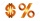What percent of 51 3/5 is 47 2/5?
• AlcoholHow many 55% alcohol we need to pour into 14 liters 75% alcohol to get p3% alcohol? How many 65% alcohol we get?
• Flags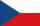How many different flags can be made from colors red, yellow, blue, green, white to consist of three different colors?
• CandiesThere are red, blue and green candies in bad. Red to green is in 6:11 ratio and blue to red in a 7: 5 ratio. In what proportion are blue to green candies?